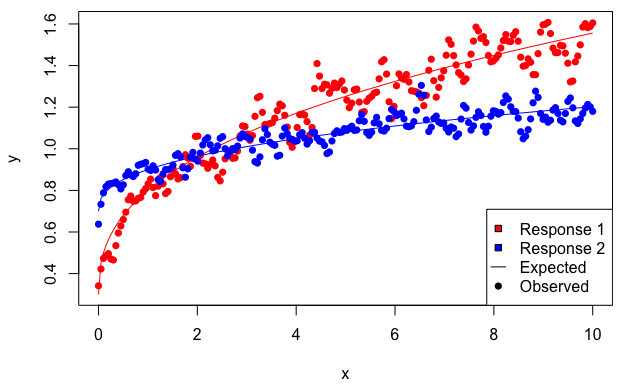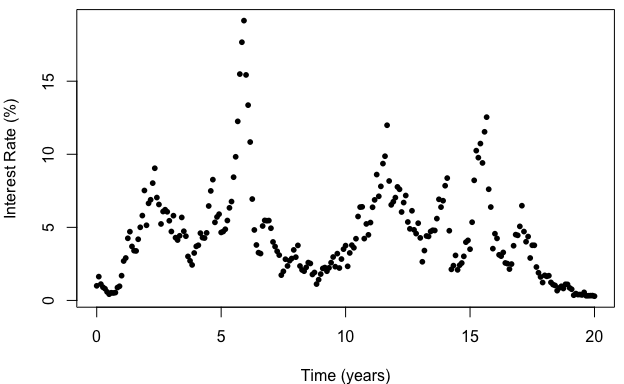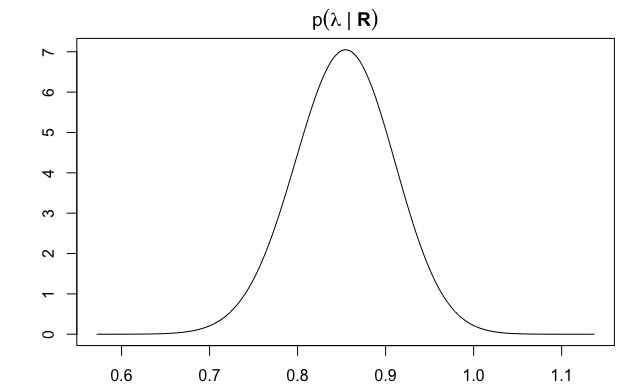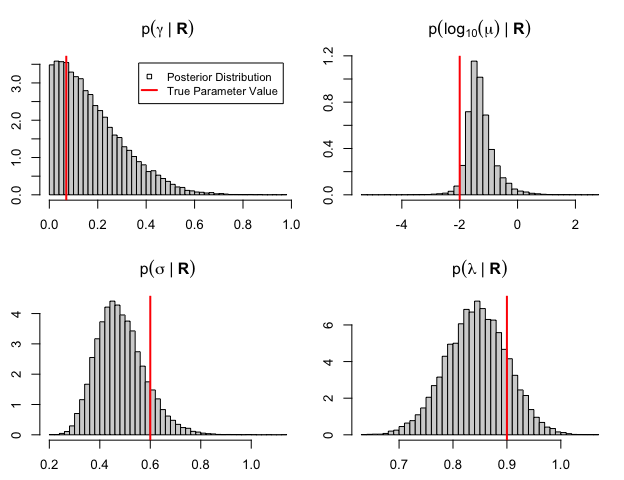# LMN: Inference for Linear Models with Nuisance Parameters

## Introduction

Consider a statistical model $$p({\boldsymbol{Y}}\mid {\boldsymbol{\theta}}, {\boldsymbol{B}}, {\boldsymbol{\Sigma}})$$ of the form $\begin{equation} {\boldsymbol{Y}}\sim \textrm{MatNorm}({\boldsymbol{X}}_{\boldsymbol{\theta}}{\boldsymbol{B}}, {\boldsymbol{V}}_{\boldsymbol{\theta}}, {\boldsymbol{\Sigma}}), \tag{1} \end{equation}$ $${\boldsymbol{X}}_{\boldsymbol{\theta}}= {\boldsymbol{X}}_{n \times p}({\boldsymbol{\theta}})$$ is the design matrix which depends on parameters $${\boldsymbol{\theta}}$$, $${\boldsymbol{B}}_{p \times q}$$ are regression coefficients, $${\boldsymbol{V}}_{\boldsymbol{\theta}}= {\boldsymbol{V}}_{n \times n}({\boldsymbol{\theta}})$$ and $${\boldsymbol{\Sigma}}_{q \times q}$$ are between-row and between-column variance matrices, and the Matrix-Normal distribution is defined as ${\boldsymbol{Z}}_{p \times q} \sim \textrm{MatNorm}({\boldsymbol{\Lambda}}_{p \times q}, {\boldsymbol{\Omega}}_{p \times p}, {\boldsymbol{\Sigma}}_{q \times q}) \quad \iff \quad \textrm{vec}({\boldsymbol{Z}}) \sim \mathcal{N}(\textrm{vec}({\boldsymbol{\Lambda}}), {\boldsymbol{\Sigma}}\otimes {\boldsymbol{\Omega}}),$ where $$\textrm{vec}({\boldsymbol{Z}})$$ is a vector stacks the columns of $${\boldsymbol{Z}}$$, and $${\boldsymbol{\Sigma}}\otimes {\boldsymbol{\Omega}}$$ denotes the Kronecker product.

Model (1) is referred to as a Linear Model with Nuisance parameters (LMN) $$({\boldsymbol{B}}, {\boldsymbol{\Sigma}})$$ for parameters of interest $${\boldsymbol{\theta}}$$. The LMN package provides tools to efficiently conduct Frequentist or Bayesian inference on all parameters $${\boldsymbol{\Theta}}= ({\boldsymbol{\theta}}, {\boldsymbol{B}}, {\boldsymbol{\Sigma}})$$ by estimating $${\boldsymbol{\theta}}$$ first, and subsequently $$({\boldsymbol{B}}, {\boldsymbol{\Sigma}})$$, as illustrated in the examples below.

## Example 1: Nonlinear Regression with Correlated Errors

Consider a toy example of the form $\begin{equation} y_{ij} = \beta_{0j} + \beta_{1j} x_i^\alpha + \varepsilon_{ij}, \tag{2} \end{equation}$ where $$i=1,\ldots,n$$, $$j=1,\ldots,q$$, and the $$\varepsilon_{ij}$$ are multivariate normal with $$E[\varepsilon_{ij}] = 0$$ and $\mathrm{cov}(\varepsilon_{ik}, \varepsilon_{jm}) = \Sigma_{ij} \times \exp\left\{-\frac{(x_k - x_m)^2}{\lambda^2}\right\}.$ Then this toy example can be written in the form of (1) with $${\boldsymbol{\theta}}= (\alpha, \lambda)$$ and \begin{aligned} {\boldsymbol{Y}}_{n\times q} & = \begin{bmatrix} y_{11} & \cdots & y_{1q} \\ \vdots & & \vdots \\ y_{n1} & \cdots & y_{nq} \end{bmatrix}, & {\boldsymbol{V}}_{n\times n}({\boldsymbol{\theta}}) & = \begin{bmatrix} e^{-\frac{(x_1-x_1)^2}{\lambda^2}} & \cdots & e^{-\frac{(x_1-x_n)^2}{\lambda^2}} \\ \vdots & & \vdots \\ e^{-\frac{(x_n-x_1)^2}{\lambda^2}} & \cdots & e^{-\frac{(x_n-x_n)^2}{\lambda^2}} \end{bmatrix}, & {\boldsymbol{X}}_{n\times 2}({\boldsymbol{\theta}}) & = \begin{bmatrix} 1 & x_1^\alpha \\ \vdots & \vdots \\ 1 & x_n^\alpha \end{bmatrix}, \\ {\boldsymbol{B}}_{2\times q} & = \begin{bmatrix} \beta_{01} & \cdots & \beta_{0q} \\ \beta_{11} & \cdots & \beta_{1q} \end{bmatrix}, & {\boldsymbol{\Sigma}}_{q\times q} & = \begin{bmatrix} \Sigma_{11} & \cdots & \Sigma_{1q} \\ \vdots & & \vdots \\ \Sigma_{q1} & \cdots & \Sigma_{qq} \end{bmatrix}. \end{aligned} Sample data is generated below with $$n = 200$$ and $$q = 2$$. Note the use of mniw::rMNorm() from the mniw package to sample from the Matrix Normal.

require(LMN)

# problem dimensions
qq <- 2 # number of responses
n <- 200 # number of observations

# parameters
Beta <- matrix(c(.3, .5, .7, .2), 2, qq)
Sigma <- matrix(c(.005, -.001, -.001, .002), qq, qq)
alpha <- .4
lambda <- .1

# simulate data
xseq <- seq(0, 10, len = n) # x vector
X <- cbind(1, xseq^alpha) # covariate matrix
V <- exp(-(outer(xseq, xseq, "-")/lambda)^2) # between-row variance
Mu <- X %*% Beta # mean matrix
Y <- mniw::rMNorm(n = 1, Lambda = Mu,
SigmaR = V, SigmaC = Sigma) # response matrix
## # response matrix
## Y <- matrix(rnorm(n*qq), n, qq)
## Y <- crossprod(chol(V), Y) %*% chol(Sigma) + Mu

# plot data
par(mfrow = c(1,1), mar = c(4,4,.5,.5)+.1)
plot(x = 0, type = "n", xlim = range(xseq), ylim = range(Mu, Y),
xlab = "x", ylab = "y")
lines(x = xseq, y = Mu[,1], col = "red")
lines(x = xseq, y = Mu[,2], col = "blue")
points(x = xseq, y = Y[,1], pch = 16, col = "red")
points(x = xseq, y = Y[,2], pch = 16, col = "blue")
legend("bottomright",
legend = c("Response 1", "Response 2", "Expected", "Observed"),
lty = c(NA, NA, 1, NA), pch = c(22, 22, NA, 16),
pt.bg = c("red", "blue", "black", "black"), seg.len = 1)Figure 1: Simulated data from the toy model (2) with $$n = 200$$ and $$q = 2$$.

The workhorse function in LMN is lmn_suff(), which calculates the sufficient statistics for $$({\boldsymbol{B}}, {\boldsymbol{\Sigma}})$$ for a given value of $${\boldsymbol{\theta}}$$.

suff <- lmn_suff(Y = Y, X = X, V = V, Vtype = "full")
sapply(suff, function(x) paste0(dim(as.array(x)), collapse = "x"))
##  Bhat     S     T   ldV     n     p     q
## "2x2" "2x2" "2x2"   "1"   "1"   "1"   "1"

Namely, the elements of suff are:

• Bhat: The $$p \times q$$ matrix $$\hat {\boldsymbol{B}}_{\boldsymbol{\theta}}= ({\boldsymbol{X}}_{\boldsymbol{\theta}}'{\boldsymbol{V}}_{\boldsymbol{\theta}}^{-1}{\boldsymbol{X}}_{\boldsymbol{\theta}})^{-1}{\boldsymbol{X}}_{\boldsymbol{\theta}}'{\boldsymbol{V}}_{\boldsymbol{\theta}}^{-1}{\boldsymbol{Y}}$$.
• T: The $$p \times p$$ matrix $${\boldsymbol{T}}_{\boldsymbol{\theta}}= {\boldsymbol{X}}_{\boldsymbol{\theta}}'{\boldsymbol{V}}_{\boldsymbol{\theta}}^{-1}{\boldsymbol{X}}_{\boldsymbol{\theta}}$$.
• S: The $$q \times q$$ matrix $${\boldsymbol{S}}_{\boldsymbol{\theta}}= ({\boldsymbol{Y}}- {\boldsymbol{X}}_{\boldsymbol{\theta}}\hat{\boldsymbol{B}}_{\boldsymbol{\theta}})'{\boldsymbol{V}}_{\boldsymbol{\theta}}^{-1}({\boldsymbol{Y}}- {\boldsymbol{X}}_{\boldsymbol{\theta}}\hat{\boldsymbol{B}}_{\boldsymbol{\theta}})$$.
• ldV: The log-determinant $$\log |{\boldsymbol{V}}_{\boldsymbol{\theta}}|$$.
• n, p, q: The dimensions of the problem.

In particular, the MLEs of $$({\boldsymbol{B}}, {\boldsymbol{\Sigma}})$$ given a particular value of $${\boldsymbol{\theta}}$$ are $$\hat {\boldsymbol{B}}_{\boldsymbol{\theta}}$$ and $$\hat {\boldsymbol{\Sigma}}_{\boldsymbol{\theta}}= {\boldsymbol{S}}_{\boldsymbol{\theta}}/n$$.

### Profile Likelihood

The profile (log)likelihood function for the LMN model corresponds to evaluating the full likelihood as a function of $${\boldsymbol{\theta}}$$ at the conditional MLE of $$({\boldsymbol{B}}, {\boldsymbol{\Sigma}})$$: $\ell_{\textrm{prof}}({\boldsymbol{\theta}}\mid {\boldsymbol{Y}}) = \ell_{\textrm{full}}({\boldsymbol{\theta}}, {\boldsymbol{B}}= \hat {\boldsymbol{B}}_{\boldsymbol{\theta}}, {\boldsymbol{\Sigma}}= \hat {\boldsymbol{\Sigma}}_{\boldsymbol{\theta}}\mid {\boldsymbol{Y}}).$ The following R code shows how to calculate the full MLE $$\hat {\boldsymbol{\Theta}}= (\hat {\boldsymbol{\theta}}, \hat {\boldsymbol{B}}, \hat {\boldsymbol{\Sigma}})$$ from only a 2D optimization of $$\ell_{\textrm{prof}}(\alpha, \lambda \mid {\boldsymbol{Y}})$$. The first step is to now that for equally-spaced $$x_i$$ as in the simulated data above, the between-row variance matrix $${\boldsymbol{V}}_{\boldsymbol{\theta}}$$ is in fact Toeplitz. It is entirely determined by its first row, and can be inverted much more efficiently than dense matrices using the R package SuperGauss.

# check than dense matrix and Toeplitz matrix calculations are the same

# autocorrelation function, or first row of V_theta
toy_acf <- function(lambda) exp(-((xseq-xseq)/lambda)^2)

# check that calculation of suff is the same
all.equal(suff,
lmn_suff(Y = Y, X = X, V = toy_acf(lambda), Vtype = "acf"))

# check that it's much faster to use Vtype = "acf"
system.time({
# using dense variance matrix
replicate(100, lmn_suff(Y = Y, X = X, V = V, Vtype = "full"))
})
system.time({
# using Toeplitz variance matrix
replicate(100, lmn_suff(Y = Y, X = X, V = toy_acf(lambda), Vtype = "acf"))
})
##  TRUE
##    user  system elapsed
##   0.103   0.010   0.113
##    user  system elapsed
##    0.03    0.00    0.03

Next, let’s find the value of the MLE $$\hat {\boldsymbol{\Theta}}$$ using stats::optim(). For display purposes the parameters will be written as ${\boldsymbol{\Theta}}= \left(\alpha, \lambda, \beta_{01}, \beta_{02}, \beta_{11}, \beta_{12}, \sigma_1 = \Sigma_{11}^{1/2}, \sigma_2 = \Sigma_{22}^{1/2}, \rho_{12} = \frac{\Sigma_{12}}{\sigma_1\sigma_2}\right).$ Also, as the SuperGauss computations require some memory allocation, we’ll declare a SuperGauss::Toeplitz() object once and re-use it within the optimization routine.

# pre-allocate memory for Toeplitz matrix calcuations
Tz <- SuperGauss::Toeplitz$new(N = n) # sufficient statistics for the toy model # sufficient statistics for toy model toy_suff <- function(theta) { X <- cbind(1, xseq^theta) Tz$set_acf(acf = toy_acf(theta))
lmn_suff(Y = Y, X = X, V = Tz, Vtype = "acf")
}

# _negative_ profile likelihood for theta
toy_prof <- function(theta) {
if(theta < 0) return(-Inf) # range restriction lambda > 0
suff <- toy_suff(theta)
-lmn_prof(suff = suff)
}

# MLE of theta
opt <- optim(par = c(alpha, lambda), # starting value
fn = toy_prof) # objective function
theta_mle <- opt$par # MLE of (Beta, Sigma) suff <- toy_suff(theta_mle) Beta_mle <- suff$Bhat
Sigma_mle <- suff$S/suff$n

# display:
# convert variance matrix to vector of standard deviations and correlations.
cov2sigrho <- function(Sigma) {
sig <- sqrt(diag(Sigma))
n <- length(sig) # dimensions of Sigma
names(sig) <- paste0("sigma",1:n)
# indices of upper triangular elements
iupper <- matrix(1:(n^2),n,n)[upper.tri(Sigma, diag = FALSE)]
rho <- cov2cor(Sigma)[iupper]
rnames <- apply(expand.grid(1:n, 1:n), 1, paste0, collapse = "")
names(rho) <- paste0("rho",rnames[iupper])
c(sig,rho)
}

Theta_mle <- c(theta_mle, t(Beta_mle), cov2sigrho(Sigma_mle))
names(Theta_mle) <- c("alpha", "lambda",
"beta_01", "beta_02", "beta_11", "beta_12",
"sigma_1", "sigma_2", "rho_12")
signif(Theta_mle, 2)
##   alpha  lambda beta_01 beta_02 beta_11 beta_12 sigma_1 sigma_2  rho_12
##   0.430   0.100   0.340   0.720   0.450   0.180   0.072   0.047  -0.370

Finally, let’s calculate standard errors for each parameter using $$\sqrt{\textrm{diag}(\widehat{\mathrm{var}}(\hat {\boldsymbol{\Theta}}))}$$, where $\widehat{\mathrm{var}}(\hat {\boldsymbol{\Theta}}) = -\left[\frac{\partial^2 \ell({\boldsymbol{\Theta}}\mid {\boldsymbol{Y}})}{\partial {\boldsymbol{\Theta}}\partial{\boldsymbol{\Theta}}'} \right]^{-1}.$ This can be done numerically using the R package numDeriv function numDeriv::hessian():

# full _negative_ loglikelihood for the toy model
toy_nll <- function(Theta) {
theta <- Theta[1:2]
Beta <- rbind(Theta[3:4], Theta[5:6])
Sigma <- diag(Theta[7:8]^2)
Sigma[1,2] <- Sigma[2,1] <- Theta*Theta*Theta
# calculate loglikelihood
suff <- toy_suff(theta)
-lmn_loglik(Beta = Beta, Sigma = Sigma, suff = suff)
}

# uncertainty estimate:
# variance estimator
Theta_ve <- solve(numDeriv::hessian(func = toy_nll, x = Theta_mle))
Theta_se <- sqrt(diag(Theta_ve)) # standard errors

# display
tab <- rbind(true = c(alpha, lambda, t(Beta), cov2sigrho(Sigma)),
mle = Theta_mle, se = Theta_se)
colnames(tab) <- paste0("$\\", gsub("([0-9]+)", "{\\1}", names(Theta_mle)), "$")
rownames(tab) <- c("True Value", "MLE", "Std. Error")
kableExtra::kable(as.data.frame(signif(tab,2)))
$$\alpha$$ $$\lambda$$ $$\beta_{01}$$ $$\beta_{02}$$ $$\beta_{11}$$ $$\beta_{12}$$ $$\sigma_{1}$$ $$\sigma_{2}$$ $$\rho_{12}$$
True Value 0.40 0.1000 0.300 0.700 0.500 0.200 0.0710 0.0450 -0.320
MLE 0.43 0.1000 0.340 0.720 0.450 0.180 0.0720 0.0470 -0.370
Std. Error 0.02 0.0012 0.038 0.022 0.031 0.015 0.0042 0.0029 0.061

## Example 2: Generalized Cox-Ingersoll-Ross Process

In Chan et al. (1992), a model for the interest rate $$R_t$$ as a function of time is given by the stochastic differential equation (SDE) $\begin{equation} \mathrm{d}R_t = -\gamma(R_t - \mu) \Delta t+ \sigma R_t^\lambda\mathrm{d}B_t, \tag{3} \end{equation}$ where $$B_t$$ is Brownian motion and the parameters are restricted to $$\gamma, \mu, \sigma, \lambda > 0$$. Suppose the data $${\boldsymbol{R}}= (R_0, \ldots, R_N)$$ consists of equispaced observations $$R_n = R_{n\cdot \Delta t}$$ with interobservation time $$\Delta t$$. A commonly-used discrete-time approximation is given by $\begin{equation} R_{n+1} \mid R_0,\ldots,R_n \sim \mathcal{N}\big(R_n - \gamma(R_n - \mu)\Delta t, \sigma^2 R_n^{2\lambda} \Delta t\big). \tag{4} \end{equation}$ Sample data from the discrete-time approximation (4) to the so-called generalized Cox-Ingersoll-Ross (gCIR) model (3) is generated below, with $$\Delta t= 1/12$$ (one month) $$N = 240$$ (20 years), and true parameter values $${\boldsymbol{\Theta}}= (\gamma, \mu, \sigma, \lambda) = (0.07, 0.01, 0.6, 0.9)$$.

# simulate data from the gcir model
gcir_sim <- function(N, dt, Theta, x0) {
# parameters
gamma <- Theta
mu <- Theta
sigma <- Theta
lambda <- Theta
Rt <- rep(NA, N+1)
Rt <- x0
for(ii in 1:N) {
Rt[ii+1] <- rnorm(1, mean = Rt[ii] - gamma * (Rt[ii] - mu) * dt,
sd = sigma * Rt[ii]^lambda * sqrt(dt))
}
Rt
}

# true parameter values
Theta <- c(gamma = .07, mu = .01, sigma = .6, lambda = .9)
dt <- 1/12 # interobservation time (in years)
N <- 12 * 20 # number of observations (20 years)

Rt <- gcir_sim(N = N, dt = dt, Theta = Theta, x0 = Theta["mu"])

par(mar = c(4,4,.5,.5))
plot(x = 0:N*dt, y = 100*Rt, pch = 16, cex = .8,
xlab = "Time (years)", ylab = "Interest Rate (%)")Figure 2: Simulated interest rates from discrete-time approximation (4) to the gCIR model (3), with $$N = 240$$, $$\Delta t= 1/12$$, and $${\boldsymbol{\Theta}}= (0.07, 0.01, 0.6, 0.9)$$.

### Bayesian Inference

The likelihood for $${\boldsymbol{\Theta}}$$ is of LMN form (1) with $${\boldsymbol{\theta}}= \lambda$$, $${\boldsymbol{B}}_{2 \times 1} = (\gamma, \gamma \mu)$$, $${\boldsymbol{\Sigma}}_{1 \times 1} = \sigma^2$$, and \begin{aligned} {\boldsymbol{Y}}_{N\times 1} & = \begin{bmatrix} R_1 - R_0 \\ \vdots \\ R_N - R_{N-1} \end{bmatrix}, & {\boldsymbol{X}}_{N \times 2} & = \begin{bmatrix} - R_0 \Delta t& \Delta t\\ \vdots & \vdots \\ - R_{N-1} \Delta t& \Delta t\end{bmatrix}, & {\boldsymbol{V}}_{N \times N}(\lambda) & = \begin{bmatrix} X_0^{2\lambda} \Delta t& & 0 \\ & \ddots & \\ 0 & & X_{N-1}^{2\lambda} \Delta t\end{bmatrix}. \end{aligned} Thus, we may proceed to maximum likelihood estimation via profile likelihood as above, using the Vtype = diag argument to lmn_suff() to optimize calculations for diagonal $${\boldsymbol{V}}$$.

# precomputed values
Y <- matrix(diff(Rt))
X <- cbind(-Rt[1:N], 1) * dt
# since Rt^(2*lambda) is calculated as exp(2*lambda * log(Rt)),
# precompute 2*log(Rt) to speed up calculations
lR2 <- 2 * log(Rt[1:N])

# sufficient statistics for gCIR model
gcir_suff <- function(lambda) {
lmn_suff(Y = Y, X = X,
V = exp(lambda * lR2) * dt, Vtype = "diag")
}

# _negative_ profile likelihood for gCIR model
gcir_prof <- function(lambda) {
if(lambda <= 0) return(Inf)
-lmn_prof(suff = gcir_suff(lambda))
}

# MLE of Theta via profile likelihood

# profile likelihood for lambda
opt <- optimize(f = gcir_prof, interval = c(.001, 10))
lambda_mle <- opt$minimum # conditional MLE for remaining parameters suff <- gcir_suff(lambda_mle) Theta_mle <- c(gamma = suff$Bhat[1,1],
mu = suff$Bhat[2,1]/suff$Bhat[1,1],
sigma = sqrt(suff$S[1,1]/suff$n),
lambda = lambda_mle)
Theta_mle
##       gamma          mu       sigma      lambda
## -0.02757594 -0.16881757  0.49526361  0.85936149

However, we can see that the profile likelihood method produces negative estimates of $$\gamma$$ and $$\mu$$, i.e., outside of the parameter support. We could try to restrict or penalize the likelihood optimization problem to obtain an admissible MLE, but then the profile likelihood simplifications would no longer apply.

Instead, consider the following Bayesian approach. First, we note that the conjugate prior for $$({\boldsymbol{B}}, {\boldsymbol{\Sigma}})$$ in the LMN model conditional on $${\boldsymbol{\theta}}$$ is the Matrix-Normal Inverse-Wishart (MNIW) distribution, {\boldsymbol{B}}, {\boldsymbol{\Sigma}}\mid {\boldsymbol{\theta}}\sim \textrm{MNIW}({\boldsymbol{\Lambda}}_{\boldsymbol{\theta}}, {\boldsymbol{\Omega}}_{\boldsymbol{\theta}}, {\boldsymbol{\Psi}}_{\boldsymbol{\theta}}, \nu_{\boldsymbol{\theta}}) \qquad \iff \qquad \begin{aligned} {\boldsymbol{B}}\mid {\boldsymbol{\Sigma}}& \sim \textrm{MatNorm}({\boldsymbol{\Lambda}}_{\boldsymbol{\theta}}, {\boldsymbol{\Omega}}_{\boldsymbol{\theta}}^{-1}, {\boldsymbol{\Sigma}}) \\ {\boldsymbol{\Sigma}}& \sim \textrm{InvWish}({\boldsymbol{\Psi}}_{\boldsymbol{\theta}}, \nu_{\boldsymbol{\theta}}), \end{aligned} where $$\textrm{InvWish}$$ denotes the Inverse-Wishart distribution, and the hyperparameters $${\boldsymbol{\Phi}}_{\boldsymbol{\theta}}= ({\boldsymbol{\Lambda}}_{\boldsymbol{\theta}}, {\boldsymbol{\Omega}}_{\boldsymbol{\theta}}, {\boldsymbol{\Psi}}_{\boldsymbol{\theta}}, \nu_{\boldsymbol{\theta}})$$ can depend on $${\boldsymbol{\theta}}$$. Thus, for the prior distribution $$\pi({\boldsymbol{B}}, {\boldsymbol{\Sigma}}, {\boldsymbol{\theta}})$$ given by

\begin{equation} \begin{aligned} {\boldsymbol{\theta}}& \sim \pi({\boldsymbol{\theta}}) \\ {\boldsymbol{B}}, {\boldsymbol{\Sigma}}\mid {\boldsymbol{\theta}}& \sim \textrm{MNIW}({\boldsymbol{\Lambda}}_{\boldsymbol{\theta}}, {\boldsymbol{\Omega}}_{\boldsymbol{\theta}}, {\boldsymbol{\Psi}}_{\boldsymbol{\theta}}, \nu_{\boldsymbol{\theta}}), \end{aligned} \tag{5} \end{equation}

we have the following analytical results:

1. The conjugate posterior distribution $$p({\boldsymbol{B}}, {\boldsymbol{\Sigma}}\mid {\boldsymbol{Y}}, {\boldsymbol{\theta}})$$ is also $$\textrm{MNIW}$$, with closed-form expressions for the hyperparameters $$\hat {\boldsymbol{\Phi}}_{\boldsymbol{\theta}}= (\hat {\boldsymbol{\Lambda}}_{\boldsymbol{\theta}}, \hat {\boldsymbol{\Omega}}_{\boldsymbol{\theta}}, \hat {\boldsymbol{\Psi}}_{\boldsymbol{\theta}}, \hat \nu_{\boldsymbol{\theta}})$$ calculated by lmn_post().

2. The marginal posterior distribution $$p({\boldsymbol{\theta}}\mid {\boldsymbol{Y}})$$ has a closed-form expression calculated by lmn_marg().

Both closed-form expressions are provided below. Putting these results together, we can efficiently conduct Bayesian inference for LMN models by first sampling $${\boldsymbol{\theta}}^{(1)}, \ldots, {\boldsymbol{\theta}}^{(B)} \sim p({\boldsymbol{\theta}}\mid {\boldsymbol{Y}})$$, then conditionally sampling $$({\boldsymbol{B}}^{(b)}, {\boldsymbol{\Sigma}}^{(b)}) \stackrel {\textrm{ind}}{\sim}\textrm{MNIW}(\hat {\boldsymbol{\Phi}}_{{\boldsymbol{\theta}}^{(b)}})$$ for $$b = 1,\ldots, B$$. This is done in the R code below with the default prior $\pi({\boldsymbol{\Theta}}) \propto |{\boldsymbol{\Sigma}}|^{-(q+1)/2},$ which is obtained from lmn_prior():

prior <- lmn_prior(p = 2, q = 1) # default prior
prior
## $Lambda ## [,1] ## [1,] 0 ## [2,] 0 ## ##$Omega
##      [,1] [,2]
## [1,]    0    0
## [2,]    0    0
##
## $Psi ## [,1] ## [1,] 0 ## ##$nu
##  0

First, we implement a grid-based sampler for $$\lambda \sim p(\lambda \mid {\boldsymbol{R}})$$:

# log of marginal posterior p(lambda | R)
gcir_marg <- function(lambda) {
suff <- gcir_suff(lambda)
post <- lmn_post(suff, prior)
lmn_marg(suff = suff, prior = prior, post = post)
}

# grid sampler for lambda ~ p(lambda | R)

# estimate the effective support of lambda by taking
# mode +/- 5 * sqrt(quadrature)
lambda_mode <- optimize(f = gcir_marg,
interval = c(.01, 10),
maximum = TRUE)$maximum lambda_quad <- -numDeriv::hessian(func = gcir_marg, x = lambda_mode) lambda_rng <- lambda_mode + c(-5,5) * 1/sqrt(lambda_quad) # plot posterior on this range lambda_seq <- seq(lambda_rng, lambda_rng, len = 1000) lambda_lpdf <- sapply(lambda_seq, gcir_marg) # log-pdf # normalized pdf lambda_pdf <- exp(lambda_lpdf - max(lambda_lpdf)) lambda_pdf <- lambda_pdf / sum(lambda_pdf) / (lambda_seq-lambda_seq) par(mar = c(2,4,2,.5)) plot(lambda_seq, lambda_pdf, type = "l", xlab = expression(lambda), ylab = "", main = expression(p(lambda*" | "*bold(R))))The grid appears to have captured the effective support of $$p(\lambda \mid {\boldsymbol{R}})$$, so we may proceed to conditional sampling. To do this effectively we use the function mniw::rmniw() in the mniw package, which vectorizes simulations over different MNIX parameters $$\hat {\boldsymbol{\Phi}}_{{\boldsymbol{\theta}}^{(1)}}, \ldots, {\boldsymbol{\Phi}}_{{\boldsymbol{\theta}}^{(1)}}$$. npost <- 5e4 # number of posterior draws # marginal sampling from p(lambda | R) lambda_post <- sample(lambda_seq, size = npost, prob = lambda_pdf, replace = TRUE) # conditional sampling from p(B, Sigma | lambda, R) BSig_post <- lapply(lambda_post, function(lambda) { lmn_post(gcir_suff(lambda), prior) }) BSig_post <- list2mniw(BSig_post) # convert to vectorized mniw format BSig_post <- mniw::rmniw(npost, Lambda = BSig_post$Lambda,
Omega = BSig_post$Omega, Psi = BSig_post$Psi,
nu = BSig_post$nu) # convert to Theta = (gamma, mu, sigma, lambda) Theta_post <- cbind(gamma = BSig_post$X[1,1,],
mu = BSig_post$X[2,1,]/BSig_post$X[1,1,],
sigma = sqrt(BSig_post\$V[1,1,]),
lambda = lambda_post)
apply(Theta_post, 2, min)
##        gamma           mu        sigma       lambda
##   -0.9897117 -717.6090519    0.2101862    0.6300930

We can see that the posterior sampling scheme above for $$p({\boldsymbol{\Theta}}\mid {\boldsymbol{R}})$$ did not always produce positive values for $$\gamma$$ and $$\mu$$. However, we can correct this post-hoc by making use of the following fact:

Rejection Sampling. Suppose that for a given prior $${\boldsymbol{\Theta}}\sim \pi({\boldsymbol{\Theta}})$$ and likelihood function $$p({\boldsymbol{R}}\mid {\boldsymbol{\Theta}})$$, we obtain a sample $${\boldsymbol{\Theta}}^{(1)}, \ldots, {\boldsymbol{\Theta}}^{(B)}$$ from the posterior distribution $$p({\boldsymbol{\Theta}}\mid {\boldsymbol{R}})$$. Then if we keep only the samples such that $${\boldsymbol{\Theta}}^{(b)} \in \mathcal{S}$$, this results in samples from the posterior distribution with likelihood $$p({\boldsymbol{R}}\mid {\boldsymbol{\Theta}})$$ and constrained prior distribution $${\boldsymbol{\Theta}}\sim \pi({\boldsymbol{\Theta}}\mid {\boldsymbol{\Theta}}\in S)$$.

In other words, if we eliminate from Theta_post all rows for which $$\gamma < 0$$ or $$\mu < 0$$, we are left with a sample form the posterior distribution with prior

$\pi({\boldsymbol{\Theta}}) \propto 1/\sigma^2 \times \boldsymbol{\textrm{I}}\{\gamma, \mu > 0\}.$

Posterior parameter distributions from the corresponding rejection sampler are displayed below.

# keep only draws for which gamma, mu > 0
ikeep <- pmin(Theta_post[,1], Theta_post[,2]) > 0
mean(ikeep) # a good number of draws get discarded
##  0.45556
Theta_post <- Theta_post[ikeep,]
# convert mu to log scale for plotting purposes
Theta_post[,"mu"] <- log10(Theta_post[,"mu"])

# posterior distributions and true parameter values
Theta_names <- c("gamma", "log(mu)", "sigma", "lambda")
Theta_true <- Theta
Theta_true["mu"] <- log10(Theta_true["mu"])
par(mfrow = c(2,2), mar = c(2,2,3,.5)+.5)
for(ii in 1:ncol(Theta_post)) {
hist(Theta_post[,ii], breaks = 40, freq = FALSE,
xlab = "", ylab = "",
main = parse(text = paste0("p(",
Theta_names[ii], "*\" | \"*bold(R))")))
abline(v = Theta_true[ii], col = "red", lwd = 2)
if(ii == 1) {
legend("topright", inset = .05,
legend = c("Posterior Distribution", "True Parameter Value"),
lwd = c(NA, 2), pch = c(22, NA), seg.len = 1.5,
col = c("black", "red"), bg = c("white", NA), cex = .85)
}
}Figure 3: Posterior distribution and true value for each gCIR parameter $${\boldsymbol{\Theta}}= (\gamma, \mu, \sigma, \lambda)$$.

## Appendix: Conjugate Posteriors for LMNs

For the regression model (1) with conjugate prior (5), the posterior distribution $$p({\boldsymbol{B}}, {\boldsymbol{\Sigma}}, {\boldsymbol{\theta}}\mid {\boldsymbol{Y}})$$ is given by

\begin{aligned} {\boldsymbol{\theta}}\mid {\boldsymbol{Y}}& \sim p({\boldsymbol{\theta}}\mid {\boldsymbol{Y}}) \propto \pi({\boldsymbol{\theta}}) \frac{\Xi({\boldsymbol{\Psi}}_{{\boldsymbol{\theta}}}, \nu_{{\boldsymbol{\theta}}})}{\Xi(\hat{\boldsymbol{\Psi}}_{{\boldsymbol{\theta}}},\hat\nu_{{\boldsymbol{\theta}}})} \left(\frac{|{\boldsymbol{\Omega}}_{{\boldsymbol{\theta}}}|(2\pi)^{-n}}{|\hat {\boldsymbol{\Omega}}_{{\boldsymbol{\theta}}}||{\boldsymbol{V}}_{{\boldsymbol{\theta}}}|}\right)^{q/2} \\ {\boldsymbol{B}}, {\boldsymbol{\Sigma}}\mid {\boldsymbol{\theta}}, {\boldsymbol{Y}}& \sim \textrm{MNIW}(\hat {\boldsymbol{\Lambda}}_{\boldsymbol{\theta}}, \hat{\boldsymbol{\Omega}}_{\boldsymbol{\theta}}, \hat {\boldsymbol{\Psi}}_{\boldsymbol{\theta}}, \hat \nu_{\boldsymbol{\theta}}), \end{aligned}

where

\begin{aligned} \Xi({\boldsymbol{\Psi}},\nu) & = \frac{|{\boldsymbol{\Psi}}|^{\nu/2}}{\sqrt{2^{\nu q}} \Gamma_q(\tfrac \nu 2)}, & \Gamma_q(a) & = \pi^{q(q-1)/4} \prod_{j=1}^q \Gamma[a + \tfrac 1 2(1-j)], \\ \hat {\boldsymbol{\Omega}}_{{\boldsymbol{\theta}}} & = {\boldsymbol{\Omega}}_{{\boldsymbol{\theta}}} + {\boldsymbol{T}}_{{\boldsymbol{\theta}}}, & \hat {\boldsymbol{\Lambda}}_{{\boldsymbol{\theta}}} & = \hat {\boldsymbol{\Omega}}_{{\boldsymbol{\theta}}}^{-1}({\boldsymbol{T}}_{{\boldsymbol{\theta}}} \hat {\boldsymbol{B}}_{{\boldsymbol{\theta}}} + {\boldsymbol{\Omega}}_{{\boldsymbol{\theta}}} {\boldsymbol{\Lambda}}_{{\boldsymbol{\theta}}}), \\ \hat \nu_{{\boldsymbol{\theta}}} & = \nu_{{\boldsymbol{\theta}}} + n, & \hat {\boldsymbol{\Psi}}_{{\boldsymbol{\theta}}} & = {\boldsymbol{\Psi}}_{{\boldsymbol{\theta}}} + {\boldsymbol{S}}_{{\boldsymbol{\theta}}} + \hat {\boldsymbol{B}}_{{\boldsymbol{\theta}}}' {\boldsymbol{T}}_{{\boldsymbol{\theta}}} \hat {\boldsymbol{B}}_{{\boldsymbol{\theta}}} + {\boldsymbol{\Lambda}}_{{\boldsymbol{\theta}}}' {\boldsymbol{\Omega}}_{{\boldsymbol{\theta}}} {\boldsymbol{\Lambda}}_{{\boldsymbol{\theta}}} - \hat {\boldsymbol{\Lambda}}_{{\boldsymbol{\theta}}}' \hat {\boldsymbol{\Omega}}_{{\boldsymbol{\theta}}} \hat {\boldsymbol{\Lambda}}_{{\boldsymbol{\theta}}}, \end{aligned}

and $${\boldsymbol{T}}_{{\boldsymbol{\theta}}}$$, $${\boldsymbol{S}}_{{\boldsymbol{\theta}}}$$ and $$\hat {\boldsymbol{B}}_{{\boldsymbol{\theta}}}$$ are outputs from lmn_suff() defined above.